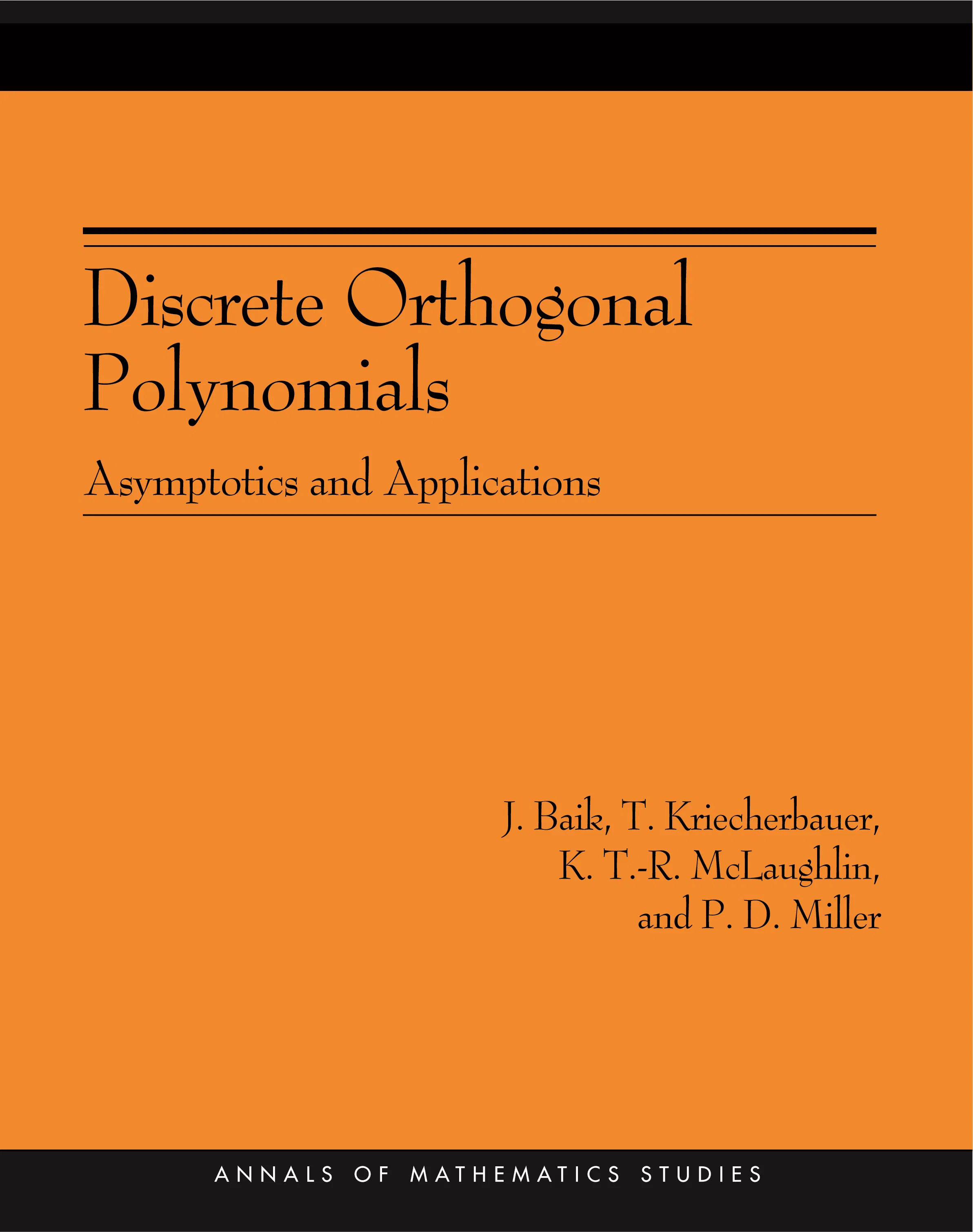#### Discrete Orthogonal Polynomials. (AM-164)

J. Baik

##### ebooks

Many of our ebooks are available for purchase from these online vendors:

Many of our ebooks are available through library electronic resources including these platforms:

# Discrete Orthogonal Polynomials. (AM-164): Asymptotics and Applications (AM-164)## Paperback

Price:
\$70.00 / £54.00
ISBN:
Published:
Jan 22, 2007
2007
Pages:
184
Size:
8 x 10 in.
Illus:
14 halftones. 6 line illus.

This book describes the theory and applications of discrete orthogonal polynomials — polynomials that are orthogonal on a finite set. Unlike other books, Discrete Orthogonal Polynomials addresses completely general weight functions and presents a new methodology for handling the discrete weights case.

J. Baik, T. Kriecherbauer, K. T.-R. McLaughlin & P. D. Miller focus on asymptotic aspects of general, nonclassical discrete orthogonal polynomials and set out applications of current interest. Topics covered include the probability theory of discrete orthogonal polynomial ensembles and the continuum limit of the Toda lattice. The primary concern throughout is the asymptotic behavior of discrete orthogonal polynomials for general, nonclassical measures, in the joint limit where the degree increases as some fraction of the total number of points of collocation. The book formulates the orthogonality conditions defining these polynomials as a kind of Riemann-Hilbert problem and then generalizes the steepest descent method for such a problem to carry out the necessary asymptotic analysis.